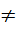# CAT 2019 Question Paper | Quants Slot 1

###### CAT Previous Year Paper | CAT Quants Questions | Question 9

Often in CAT Question Paper, we come across questions which appear easy but are actually curveballs. This is one such question. It appears very easy but is likely to consume a lot of time while the accuracy levels may be low. Questions like this separate the ones who have done their CAT online preparation vis-a-vis the folks who have just appeared after seeing the CAT syllabus. Please solve this question carefully.

.

Question 9 : For any positive integer n, let f(n) = n(n + 1) if n is even, and f(n) = n + 3 if n is odd. If m is a positive integer such that 8f(m + 1) - f(m) = 2, then m equals [TITA]

## Best CAT Coaching in Chennai

#### CAT Coaching in Chennai - CAT 2022Limited Seats Available - Register Now!

If n is even, f(n) = n (n + 1)
So, f (2) = 2(2+1) = 2 (3) = 6
If n is odd, f(n) = n + 3
f (1) = 1 + 3 = 4
It is given that, 8 x f(m+1) - f(m) =2
So, m can either be even or odd
Case-1: If m were even and m+1 odd
So, 8 x f(m+1) - f(m) =2
8(m + 4) - m (m + 1) = 2
8m + 32 - m2 - m = 2
m2 - 7m - 30 = 0
(m-10) (m+3) = 0
m = 10 or -3
m = 10, since m is positive
Case-2: If m were even and m+1 odd
8 x f(m+1) - f(m) =2
8 (m +1) (m + 2) - (m + 3) = 2
Now, let us substitute m = 1 which is the minimum possible value
8 (1 + 1) (1 + 2) - (1 + 3)3
Case 2 does not work

The question is "For any positive integer n, let f(n) = n(n + 1) if n is even, and f(n) = n + 3 if n is odd. If m is a positive integer such that 8f(m + 1) - f(m) = 2, then m equals [TITA]"

##### Hence, the answer is 10

###### CAT Coaching in ChennaiCAT 2023

Classroom Batches Starting Now! @Gopalapuram

###### Best CAT Coaching in Chennai Introductory offer of 5000/-

Attend a Demo Class

##### Where is 2IIM located?

2IIM Online CAT Coaching
A Fermat Education Initiative,
58/16, Indira Gandhi Street,
Kaveri Rangan Nagar, Saligramam, Chennai 600 093

##### How to reach 2IIM?

Mobile: (91) 99626 48484 / 94459 38484
WhatsApp: WhatsApp Now
Email: info@2iim.com